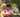### MinPerimeterRectangle

February 13, 2020

MinPerimeterRectangle

An integer N is given, representing the area of some rectangle.
The area of a rectangle whose sides are of length A and B is A B, and the perimeter is 2 (A + B). The goal is to find the minimal perimeter of any rectangle whose area equals N. The sides of this rectangle should be only integers.

For example, given integer N = 30, rectangles of area 30 are:
• (1, 30), with a perimeter of 62,
• (2, 15), with a perimeter of 34,
• (3, 10), with a perimeter of 26,
• (5, 6), with a perimeter of 22.

Write a function:
function solution(N);
that, given an integer N, returns the minimal perimeter of any rectangle whose area is exactly equal to N. For example, given an integer N = 30, the function should return 22, as explained above.

Write an efficient algorithm for the following assumptions:
• N is an integer within the range [1..1,000,000,000].

``````function solution(N) {
// write your code in JavaScript (Node.js 8.9.4)
const n = Math.floor( Math.sqrt(N) )
let min = Infinity
let p
for(let i=1; i<=n; i++){
if(N%i==0) { // remainder=0
p = 2*(i+N/i)
if( p<min ) min = p
}
}

return min
}``````Posted by Tai Lu ( 呂台生 ) who's building useful things.You should follow him on Twitter